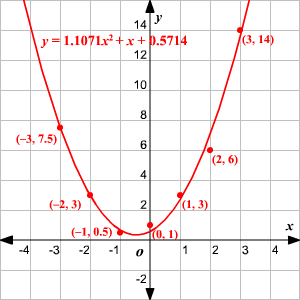A quadratic regression is the process of finding the equation of the parabola that best fits a set of data. As a result, we get an equation of the form:

$y=a{x}^{2}+bx+c$ where $a\ne 0$ .

The best way to find this equation manually is by using the least squares method. That is, we need to find the values of $a,b,\text{\hspace{0.17em}}\text{\hspace{0.17em}}\text{and}\text{\hspace{0.17em}}\text{\hspace{0.17em}}c$ such that the squared vertical distance between each point $\left({x}_{i},{y}_{i}\right)$ and the quadratic curve $y=a{x}^{2}+bx+c$ is minimal.

The matrix equation for the quadratic curve is given by:

$\left[\begin{array}{ccc}\sum {x}_{i}{}^{4}& \sum {x}_{i}{}^{3}& \sum {x}_{i}{}^{2}\\ \sum {x}_{i}{}^{3}& \sum {x}_{i}{}^{2}& \sum {x}_{i}\\ \sum {x}_{i}{}^{2}& \sum {x}_{i}& n\end{array}\right]\left[\begin{array}{c}a\\ b\\ c\end{array}\right]=\left[\begin{array}{c}\sum {x}_{i}{}^{2}{y}_{i}\\ \sum {x}_{i}{y}_{i}\\ \sum {y}_{i}\end{array}\right]$

The relative predictive power of a quadratic model is denoted by  ${R}^{2}$ .

This can be obtained using the formula:

${R}^{2}=1-\frac{\text{SSE}}{\text{SST}}$ where $\text{SSE}=\sum {\left({y}_{i}-a{x}_{i}{}^{2}-b{x}_{i}-c\right)}^{2}$ and $\text{SST}={\sum \left({y}_{i}-\stackrel{¯}{y}\right)}^{2}$

The value of  ${R}^{2}$  varies between $0$ and $1$ . The closer the value is to $1$ , the more accurate the model is.

But these are very tedious calculations. So, we will use a graphing calculator to automatically calculate the curve.

Example 1:

Consider the set of data. Determine the quadratic regression for the set.

$\left(-3,7.5\right),\left(-2,3\right),\left(-1,0.5\right),\left(0,1\right),\left(1,3\right),\left(2,6\right),\left(3,14\right)$

Enter the $x$ -coordinates and $y$ -coordinates in your calculator and do a quadratic regression. The equation of the parabola that best approximates the points is

$y=1.1071{x}^{2}+x+0.5714$

Plot the graph. You should get a graph like this.You can see that the ${R}^{2}$ value for the data is $0.9942$ .